Courses

# Environmental Engineering 4

## 10 Questions MCQ Test RRB JE for Civil Engineering | Environmental Engineering 4

Description
This mock test of Environmental Engineering 4 for Civil Engineering (CE) helps you for every Civil Engineering (CE) entrance exam. This contains 10 Multiple Choice Questions for Civil Engineering (CE) Environmental Engineering 4 (mcq) to study with solutions a complete question bank. The solved questions answers in this Environmental Engineering 4 quiz give you a good mix of easy questions and tough questions. Civil Engineering (CE) students definitely take this Environmental Engineering 4 exercise for a better result in the exam. You can find other Environmental Engineering 4 extra questions, long questions & short questions for Civil Engineering (CE) on EduRev as well by searching above.
QUESTION: 1

### Arrange the given lakes in the descending order of their productivity level Mesotrophic Lakes Oligotrophic Lakes Eutrophic Lakes

Solution:

Eutrophic Lakes: These are the lakes of high productivity level, hence support high growth of algae.

Mesotrophic Lakes: These are the lakes of intermediate productivity level, hence support intermediate growth of algae.

Oligotrophic Lakes: These are the lakes of least productivity level, hence support negligible growth of algae.

QUESTION: 2

### The following zones are formed in a polluted river. (1) zone of clear water (2) zone of active decomposition (3) zone of recovery (4) zone of pollution The correct sequence in which these zones occur progressively downstream in a polluted river is

Solution: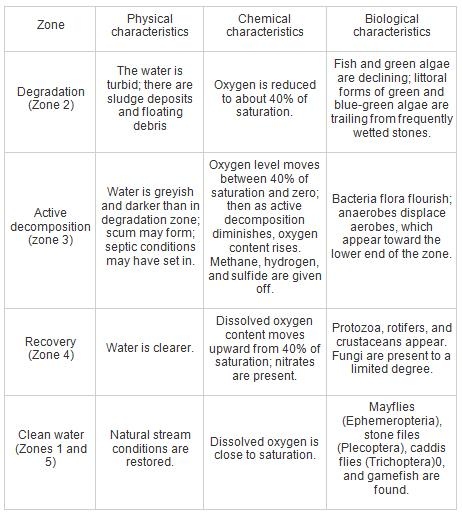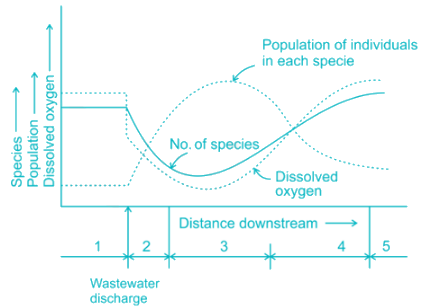QUESTION: 3

### In conventional activates sludge process, the mean cell residence time in the aeration tank is–

Solution:

Mean cell residence time in activated sludge process in aeration tank is in range of 4 to 15 days.

QUESTION: 4

The ultimate BOD value of a waste -

Solution:

The ultimate BOD value of waste is the amount of biodegradable organic matter presents in the sewage sample. At any temperature the amount of organic matter present does not change, so the ultimate BOD will remain same for any temperature.

*Answer can only contain numeric values
QUESTION: 5

Deoxygenation constant [kD] at 40o C ----- per day. Assume kD at 20o C is 0.13 per day.

Solution:

[kD]To= [kD]20o[1.047]T - 20

[kD]40oC = 0.13 [1.047]40 - 20

[kD]40oC = 0.13 [1.047]20

[kD]40oC = 0.326 per day

QUESTION: 6

The technique utilized for the disposal of biomedical waste​, in which it is in contact with steam under controlled pressure and temperature condition with the end goal to complete sterilization is

Solution:

Autoclaving:- The method used for the disposal of biomedical waste is , in which it is brought in closed contact with steam under controlled pressure and temperature condition in order to carry out disinfection.

Pulverization and Shredding: Basically this is not a method of disposal but in these method heavier solids are broken into the lighter one either by cutting action or by grinding action.

Pulverization refers to the action of crushing and grinding, whereas shredding refers to the action of cutting and tearing.

Pyrolysis: is a method of disposal of the refuse in which burning of refuse is generally done at high temperature in the almost absence of oxygen.

QUESTION: 7

The ultimate BOD of wastewater is 300 mg/L and the reaction rate constant (to the base ‘e’) at 20°C is 0.3585/day, then the 5 days BOD at 20oC will be.

Solution:

Concept:

Ultimate BOD = Lo

BOD5­ = 5day BOD, of water/waste water

BOD at any time ‘t’ is (Lt)
Lt = Lo(1 − e−kDt)

Where Lo = ultimate BOD

KD = Rate constant

Calculation:

Lt = 300 × (1 – e-0.3585 × t)

L5 = BOD5 = 300 × (1 – e-0.3585 × 5)

= 250 mg/L

*Answer can only contain numeric values
QUESTION: 8

The percentage of the relative stability of a test conducted for the determination of relative conductivity at 20°C when the incubation was done for 20 days is _____

Solution:

Relative stability (Sr) at 20°C
Sr = 100 [1 − (0.794)t]
= 100 [1− (0.794)20]
= 99.01%

*Answer can only contain numeric values
QUESTION: 9

A domestic sewage has a BOD of 90 gm per capita. The waste is flowing at a rate of 0.9 MLD having a BOD of 200 mg/l. The population equivalent of the waste is _____.

Solution:

Q = 0.9 MLD

5 day BOD, Y5 = 200 mg/L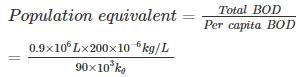= 2000

QUESTION: 10

A large stream has a reoxygenation constant of 0.45 per day and at a point at which organic pollutant is discharged, it is saturated with oxygen at 12 mg/L (Do = 0). Below the outfall, the ultimate demand for oxygen is found to be 19 mg/l and the Deoxygenation Constant is 0.2 per day. Calculate, the D.O at 36 km downstream Assume velocity of the stream to be 1.2 m/s.

Solution:

Time required for certain amount of D.O at 36 km downstream is given by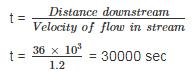t = 0.3472 days

Oxygen deficit after time t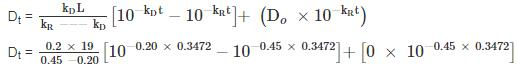Dt = 2.35 mg/l

D.O at 36 km downstream = 12 - 2.35

= 9.65 mg/l